# Resources tagged with: GeoGebra

Filter by: Content type:
Age range:
Challenge level:

### There are 90 results

Broad Topics > Physical and Digital Manipulatives > GeoGebra##### Age 14 to 16 Challenge Level:

Make five different quadrilaterals on a nine-point pegboard, without using the centre peg. Work out the angles in each quadrilateral you make. Now, what other relationships you can see?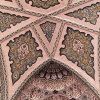### Angles Inside

##### Age 11 to 14 Challenge Level:

Draw some angles inside a rectangle. What do you notice? Can you prove it?### Semi-regular Tessellations

##### Age 11 to 16 Challenge Level:

Semi-regular tessellations combine two or more different regular polygons to fill the plane. Can you find all the semi-regular tessellations?### Right Angles

##### Age 11 to 14 Challenge Level:

Can you make a right-angled triangle on this peg-board by joining up three points round the edge?### Subtended Angles

##### Age 11 to 14 Challenge Level:

What is the relationship between the angle at the centre and the angles at the circumference, for angles which stand on the same arc? Can you prove it?### Triangles in Circles

##### Age 11 to 14 Challenge Level:

Can you find triangles on a 9-point circle? Can you work out their angles?### Tessellation Interactivity

##### Age 7 to 16 Challenge Level:

An environment that enables you to investigate tessellations of regular polygons##### Age 11 to 16 Challenge Level:

Draw some quadrilaterals on a 9-point circle and work out the angles. Is there a theorem?### Polygon Rings

##### Age 11 to 14 Challenge Level:

Join pentagons together edge to edge. Will they form a ring?### Bow Tie

##### Age 11 to 14 Challenge Level:

Show how this pentagonal tile can be used to tile the plane and describe the transformations which map this pentagon to its images in the tiling.### The Medieval Octagon

##### Age 14 to 16 Challenge Level:

Medieval stonemasons used a method to construct octagons using ruler and compasses... Is the octagon regular? Proof please.### Spinners Environment

##### Age 5 to 18 Challenge Level:

A tool for generating random integers.### Using Geogebra

##### Age 11 to 18### Same Length

##### Age 11 to 16 Challenge Level:

Construct two equilateral triangles on a straight line. There are two lengths that look the same - can you prove it?### Estimating Angles

##### Age 7 to 14 Challenge Level:

How good are you at estimating angles?### Where Is the Dot?

##### Age 14 to 16 Challenge Level:

A dot starts at the point (1,0) and turns anticlockwise. Can you estimate the height of the dot after it has turned through 45 degrees? Can you calculate its height?### Rolling Around

##### Age 11 to 14 Challenge Level:

A circle rolls around the outside edge of a square so that its circumference always touches the edge of the square. Can you describe the locus of the centre of the circle?### Points in Pairs

##### Age 14 to 16 Challenge Level:

Move the point P to see how P' moves. Then use your insights to calculate a missing length.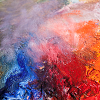### Mixing More Paints

##### Age 14 to 16 Challenge Level:

Can you find an efficent way to mix paints in any ratio?### Pythagoras Proofs

##### Age 14 to 16 Challenge Level:

Can you make sense of these three proofs of Pythagoras' Theorem?### L-triominoes

##### Age 14 to 16 Challenge Level:

L triominoes can fit together to make larger versions of themselves. Is every size possible to make in this way?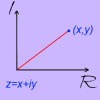### A Brief Introduction to the Argand Diagram

##### Age 14 to 18 Challenge Level:

Complex numbers can be represented graphically using an Argand diagram. This problem explains more...### Mixing Paints

##### Age 11 to 14 Challenge Level:

Can you work out how to produce different shades of pink paint?##### Age 14 to 16 Challenge Level:### Nine Colours

##### Age 11 to 16 Challenge Level:

Can you use small coloured cubes to make a 3 by 3 by 3 cube so that each face of the bigger cube contains one of each colour?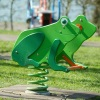### Surprising Equalities

##### Age 14 to 18 Challenge Level:

Take any triangle, and construct squares on each of its sides. What do you notice about the areas of the new triangles formed?### Polar Coordinates

##### Age 14 to 18

Cartesian Coordinates are not the only way!### Colour in the Square

##### Age 7 to 16 Challenge Level:

Can you put the 25 coloured tiles into the 5 x 5 square so that no column, no row and no diagonal line have tiles of the same colour in them?### Squirty

##### Age 14 to 16 Challenge Level:

Using a ruler, pencil and compasses only, it is possible to construct a square inside any triangle so that all four vertices touch the sides of the triangle.### Tilting Triangles

##### Age 14 to 16 Challenge Level:

A right-angled isosceles triangle is rotated about the centre point of a square. What can you say about the area of the part of the square covered by the triangle as it rotates?### Vanishing Point

##### Age 14 to 18 Challenge Level:

How can visual patterns be used to prove sums of series?### Robotic Rotations

##### Age 11 to 16 Challenge Level:

How did the the rotation robot make these patterns?### Squaring the Circle and Circling the Square

##### Age 14 to 16 Challenge Level:

If you continue the pattern, can you predict what each of the following areas will be? Try to explain your prediction.### Strolling Along

##### Age 14 to 18 Challenge Level:

What happens when we multiply a complex number by a real or an imaginary number?### Opening the Door

##### Age 14 to 18 Challenge Level:

What happens when we add together two complex numbers?### Into the Wilderness

##### Age 14 to 18 Challenge Level:

Let's go further and see what happens when we multiply two complex numbers together!### Mapping the Territory

##### Age 14 to 18 Challenge Level:

Can you devise a system for making sense of complex multiplication?##### Age 14 to 16 Challenge Level:

The points P, Q, R and S are the midpoints of the edges of a non-convex quadrilateral.What do you notice about the quadrilateral PQRS and its area?### More Twisting and Turning

##### Age 11 to 16 Challenge Level:

It would be nice to have a strategy for disentangling any tangled ropes...### Beelines

##### Age 14 to 16 Challenge Level:

Is there a relationship between the coordinates of the endpoints of a line and the number of grid squares it crosses?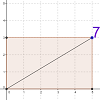### Exploring Diagonals

##### Age 11 to 16

Move the corner of the rectangle. Can you work out what the purple number represents?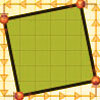### At Right Angles

##### Age 14 to 16 Challenge Level:

Can you decide whether two lines are perpendicular or not? Can you do this without drawing them?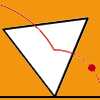### Speeding Up, Slowing Down

##### Age 11 to 14 Challenge Level:

Experiment with the interactivity of "rolling" regular polygons, and explore how the different positions of the red dot affects its speed at each stage.### Solving Together - Estimating Angles

##### Age 11 to 14

Week 2
How well can you estimate angles? Playing this game could improve your skills.### Square Coordinates

##### Age 11 to 14 Challenge Level:

A tilted square is a square with no horizontal sides. Can you devise a general instruction for the construction of a square when you are given just one of its sides?### Reflecting Squarely

##### Age 11 to 14 Challenge Level:

In how many ways can you fit all three pieces together to make shapes with line symmetry?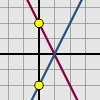### Reflecting Lines

##### Age 11 to 14 Challenge Level:

Investigate what happens to the equations of different lines when you reflect them in one of the axes. Try to predict what will happen. Explain your findings.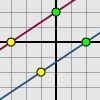### Translating Lines

##### Age 11 to 14 Challenge Level:

Investigate what happens to the equation of different lines when you translate them. Try to predict what will happen. Explain your findings.### Just Rolling Round

##### Age 14 to 16 Challenge Level:

P is a point on the circumference of a circle radius r which rolls, without slipping, inside a circle of radius 2r. What is the locus of P?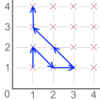### Coordinates of Corners

##### Age 11 to 16

Use the applet to make some squares. What patterns do you notice in the coordinates?